An illustration of The Leaning Tower of Teluk Intan, Perak, Malaysia

# Clustering

Clustering is categorized under unsupervised learning, which forms the niche part of machine learning. Unlike supervised learning which is more common in most common machine learning study, classification tasks learn from the provided labeled data and makes class predictions. However, this does not cause the clustering method to be less desirable, as clustering algorithms are essential in discovering unexplored insights. Thus, it is important to understand the performance of the clustering task and to decide whether the clusters formed are trustable.

# Silhouette Analysis

There are various performance metrics that you can implement for your clustering studies, namely:

• Silhouette Analysis
• Rand Index
• Mutual Information
• Calinski-Harabasz Index
• Davies-Bouldin Index

Silhouette Analysis is the most common method as it is more straightforward compared to others. Silhouette Analysis or Silhouette Plot is often used with the KMeans algorithm to measure the separation distance between clusters. The concept is based on evaluating the performance of the model itself instead of the results, where the ground truth label is unknown. It exhibits the nature of the clusters formed, by how close they are within the range of [-1,1].

A silhouette score of “+1” indicates that a specific data point is distant away from its neighboring cluster and very close to the cluster group it is assigned. In contrast, a value of “-1” indicates that the point is close to its neighboring cluster compared to the cluster it is assigned. As for the value of “0”, it means the data point most likely lies at the boundary of the distance between the two clusters. Value of “+1” is the ideal score to achieve to have a good clustering performance whereas “-1” is least preferred. However, a silhouette score of “+1” is seemingly hard to achieve in real life, when dealing with unstructured and complex data. Hence, it can be deduced that the higher the silhouette score is within a study scenario, the better the configuration is, but assuming the best scores are at least higher than 0.

Diving deeper into the mathematical derivation, the silhouette score is calculated using the mean intra-cluster distance, a, and the mean nearest-cluster distance, b for each sample, with a condition where the number of labels to be at least larger than 2 and smaller than the number of samples. (If the number of sample points is 100, the number of labels/clusters can be at most 99, such that the clustering will not end up with one point in one cluster. However, having 99 clusters among 100 sample points is not realistic as well.)

`Silhouette coefficient = (b-a)/ max(a,b)** 2 < n_labels < n_sample_points - 1`

The figure above shows a typical silhouette plot, where the x-axis represents the cluster label and the y-axis represents the silhouette coefficient/score. How do we know if a specific number of clusters is to be chosen among a range of cluster numbers after trying out? By averaging the Silhouette coefficient, a global/average silhouette score can be computed (marked as a red dotted line) into a single value which serves as a benchmark for evaluating the overall performance. Therefore, one should pick the clusters which have coefficient values exceeding the average silhouette score. Another key parameter to look out for when interpreting the plot is, the thickness of each cluster is to be as uniform as possible, to eliminate the possibility of poor clustering groups.

# Example

Normally, the silhouette plot is plotted not just for one cluster number instead, a range of acceptable values based on the number of data points. For starters, many Python libraries help to visualize the clustering results, such as YellowBricks Library.

The code below shows an example of using Python code with YellowBricks Silhouette Visualizer to showcase the clustering result.

`from sklearn.cluster import KMeansfrom yellowbrick.cluster import SilhouetteVisualizer** scaled_data here represents collection of data composed using pandas dataframe** Section 1cluster_nums = list(range(2, 10))scores = []for cluster_num in cluster_nums:  kmeans = KMeans(n_clusters=cluster_num, random_state=42)  kmeans.fit(scaled_data)  clusters = kmeans.predict(scaled_data)  silhouette = silhouette_score(scaled_data, clusters)  scores.append(silhouette)plt.title("Silhouette Score for cluster n=2-10")plt.ylabel('Silhouette Score')plt.xlabel('Clusters')plt.figure(1)plt.plot(cluster_nums, scores)** Section 2fig, ax = plt.subplots(2, 2, figsize=(15, 8))for i in [2, 3, 4, 5]:  # Create KMeans instance for different number of clusters  km = KMeans(n_clusters=i, init='k-means++',  n_init=10, max_iter=500, random_state=42)  q, mod = divmod(i, 2)  # Create SilhouetteVisualizer instance with KMeans instance  # Fit the visualizer  visualizer = SilhouetteVisualizer(  km, colors='yellowbrick', ax=ax[q-1][mod])  visualizer.fit(scaled_data)fig.suptitle("Silhouette plot for clusers n=2-5")ax[0, 0].title.set_text('Number of clusters = 2')ax[0, 1].title.set_text('Number of clusters = 3')ax[1, 0].title.set_text('Number of clusters = 4')ax[1, 1].title.set_text('Number of clusters = 5')plt.show()`

Section 1 shows how the average silhouette score is plotted for cluster numbers ranging from 2–10. The relationship can be deduced as a positive one as the silhouette score improves with the increment of clusters. As for Section 2 of the code, only 4 of the clusters evaluated using KMeans are shown below:

The y-axis here represents the individual sample points ordered by cluster group. Overall, the number of clusters of 2, 3, 4, and 5 are not considered as sub-optimal as not all of the silhouette scores of the clusters are larger than the average silhouette coefficient. Not only that, it seems that the thickness of one cluster is always comparatively larger than the others, which results in two possibilities: either the data columns of the sample points are not well defined, or the cluster number used for KMeans still needed to be increased or refined. However, a rule of thumb is one should not increase the number of clusters just to achieve the highest silhouette score as this is considered as computing inefficiency, as increasing the resources used is no longer getting back the expected performance.

# Conclusion

In short, performance metrics play an important role in machine learning, especially for unsupervised learning studies. This helps the researchers to understand more on how they should proceed, or how can they improve the performance by data-processing and feature-refining, depending on the results shown from the analysis.

XIMNET is a digital solutions provider with two decades of track records specialising in web application development, AI Chatbot and system integration.

XIMNET is launching a brand new way of building AI Chatbot with XYAN. Get in touch with us to find out more.

--

--

--

## More from ximnet

Digital Agency in Malaysia | www.ximnet.com.my

Love podcasts or audiobooks? Learn on the go with our new app.

## Whose Line Is It Anyway?## PyTorch: Directly use pre-trained AlexNet for Image Classification and Visualization of the…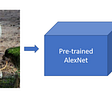## Build High Quality Training Data for Computer Vision and NLP Applications## Introduction To Machine Learning!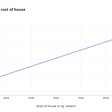## ShaRF: Take a Picture From a Real-Life Object, and Create a 3D Model of It## The Million-Dollar Neural Network, Part II: Machine Learning Intuition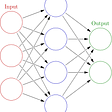## Intro to TensorFlow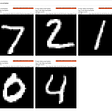## ximnet

Digital Agency in Malaysia | www.ximnet.com.my

## Grouping Districts and Cities in East Java Based on Its Economic Inequality Metrics Through the…## Dynamic Causal Modelling for EEG and MEG¹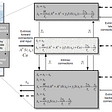## Types of Machine Learning